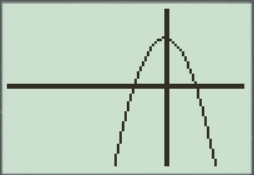# To graph : The quadratic equation y = 256 − x 2 4 in a graphing window.### Precalculus: Mathematics for Calcu...

6th Edition
Stewart + 5 others
Publisher: Cengage Learning
ISBN: 9780840068071### Precalculus: Mathematics for Calcu...

6th Edition
Stewart + 5 others
Publisher: Cengage Learning
ISBN: 9780840068071

#### Solutions

Chapter 1.9, Problem 15E
To determine

## To graph: The quadratic equation y=256−x24 in a graphing window.

Expert Solution

### Explanation of Solution

Given information:

Graph:

The graph of the quadratic equation y=256x24 can be sketched in the cartesian plane,

Consider the quadratic equation, y=256x24 .

Now put the values of x in the equation to find the values of y or to find the real roots of y with the help of x .

Rewrite the equation:

y=256x214

Take power 4 both sides:

y=256x214y4=256x2(y2)2+x2=256(y2)2+x2=162

Compare this (y2)2+x2=162 equation with x2+y2=16 .

substitute the value of x=0 in equation y=256x24 :

y=256x24y=25604y=444y=4

Observe that as the value of x increases there is slightly increment in value of y .

Steps to plot the graph of the equation y=256x24 with the help of graphing utility are as follows:

Step 1: Press MODE key.

Step 2: Use the down arrow key to reach FUNC option.

Step 3: Press ENTER key.

Step 4: Press Y= key.

Step 5: Enter the function 256x24 .

Step 6: Press WINDOW key. Change the settings to

Xmin=20Xmax=10Ymin=10Ymax=20

For better view of graph.

Step 8: Press GRAPH key.

The result obtained on the screen is provided below,Interpretation:

The equation of the function y=256x24 represents a parabola.

The parabola opens downwards.

The x intercepts are the points on xaxis where the graph of the equation touches xaxis .

At x -axis y is always zero.

Recall that the graphical approach to solve the equation simultaneously.

Therefore, in the equation, y=256x24 there is no x intercepts.

Therefore, the equation y=256x24 is symmetric about the y axis Initially the graph of the function y=256x24 increases when increase the value of x ,the graph is a parabola shaped graph.

### Have a homework question?

Subscribe to bartleby learn! Ask subject matter experts 30 homework questions each month. Plus, you’ll have access to millions of step-by-step textbook answers!